# Current Density in Metal and Semiconductor

The concept of current density is very simple but it is much use full in the field of electrical engineering.

## Current Density in Metal

Suppose, we have taken one conductor of cross-section 2.5 square mm. Now due to applied electric potential, the conductor carries a current of 3 A. Now the electric current carried by the conductor per square mm of its cross-section is 3/2.5 = 1.2 A. Here we considered that the current in the conductor is uniformly distributed throughout its cross-section. Now this 1.2 ampere is the current density of the conductor, and obviously, it is measured in ampere per square millimetre or more theoretically ampere per unit area. Hence, the current density can be defined as the electric current carried by conductor per unit cross-sectional area of the conducting medium.

We denote the current density with J and J equals I/A. Here, ‘I’ is the uniformly distributed current being carried by the conductor of the cross-sectional area A. If total N number of electrons passing through a cross-section of a conductor in time T, then Ne is the charge transferring through this cross-section in same time T. Where e is the charge of an electron in coulomb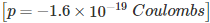Now the amount of charge passing the cross-section per unit time is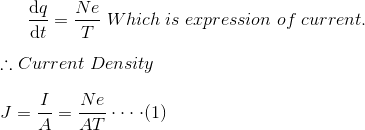Again if N number of electrons lie in the L length of the conductor, then the electron concentration is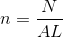Now, from equation (1) we can write,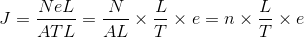Since, N number of electrons lie in the length L and they all pass the cross-section in time T, the drift velocity of the electrons will be,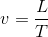Hence, equation (2) can also be rewritten as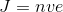Now if applied electric field to the conductor is E, then drift velocity of the electrons increases proportionally,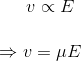Where, μ is defined as the mobility of electrons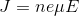## Current Density of Semiconductors

For calculating current density of semiconductor, some factors to be considered.

1. In semiconductor current flows not only due to electrons instead it is due to drift of electrons as well as holes.
2. Movement of holes is always in opposite to that of corresponding electrons.
3. Holes contribute current to their direction of movement whereas electrons contribute current opposite to their direction of movement. Hence both currents will be in same direction.
4. Electrons involved in causing current in semiconductor, move through conduction band whereas holes causing current in semiconductor move through valance band. That is why mobility of electrons and holes are different in semiconductor.

Current density in semiconductor will be,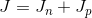Where, Jn is the current density due to mobile electrons.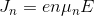Where, Jp is the current density due to mobile holes,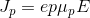Then,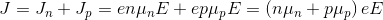Where, n and p are the concentration of mobile electrons and holes respectively, e is absolute charge of each electron and hole and μn and μp are mobility of electrons and holes respectively.

### What is the Relation Between Current Density and Conductivity?

The expression of current density is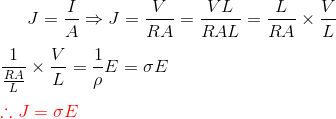Where, V is the applied voltage across conductor,
R is the resistance of the conductor,
A is the cross-sectional area of the conductor,
L is the length of the conductor,
ρ is the resistivity of the conductor,
σ (= 1/ρ) is the conductivity of the conductor.
E is the electric field intensity voltage/length.

Want To Learn Faster? 🎓
Get electrical articles delivered to your inbox every week.
No credit card required—it’s 100% free.